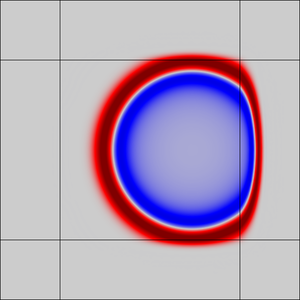# Application Gallery

#### Gaussian Pulse in 2D Uniform Flow: Convected Wave Equation and Absorbing Layers

Application ID: 36241

This tutorial simulates a standard test and benchmark model for nonreflecting conditions and sponge layers for linearized Euler-like systems. It involves the propagation of a transient Gaussian pulse in a 2D uniform flow. The Convected Wave Equation, Time Explicit interface solves the linearized Euler equations with an adiabatic equation of state and the interface uses the Absorbing Layers feature to model infinite domains.

An acoustic pulse is generated by an initial Gaussian distribution at the center of the computational domain. The pulse propagates in a high Mach number uniform flow. The analytical solution to the problem is used to validate the solution and it shows very good agreement.

The model also illustrates how to set up and use the absorbing layers. By using absorbing layers, you can reduce spuriously reflected waves to 1/1000 of the incident field amplitude.This model example illustrates applications of this type that would nominally be built using the following products: